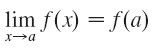# Stewart – Calculus – 2.5 – Continuity

1. Definition: A function f is continuous at a number a if

This definition implicitly requires three things if f is continuous at a:

1. f(a) is defined (that is, a is in the domain of f)

2.$\lim_{x \to a}f(x)$ exists

3.$\lim_{x \to a}f(x)=f(a)$

2. If$f$ and$g$ are continuous functions with$f(3)=5$ and$\lim_{x \to 3}[2f(x) - g(x)]=4$, find$g(3)$.

Use the definition of continuity and the properties of limits to show that the funtion is continous at the given number a.$f(x)=(x+2x^{3})^{4}$,$a=-1$

3. The Intermediate Value Theorem
Suppose that$f$ is continuous on the closed interval$[a, b]$ and let$N$ be any number between$f(a)$ and$f(b)$, where$f(a) \neq f(b)$. Then there exists a number$c$ in$(a,b)$ such that$f(c)=N$.

~ ~ ~

Use the Intermediate Value Theorem to show that there is
a root of the given equation in the specified interval.$x^4+x-3=0, (1,2)$